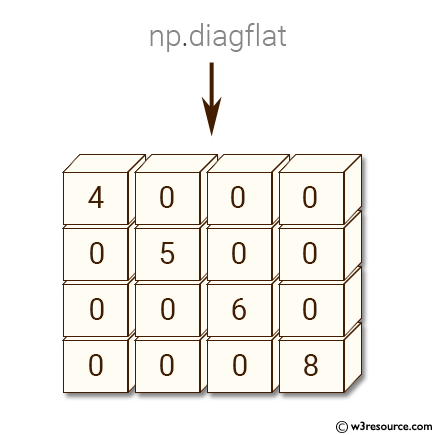﻿ NumPy: Create a 2-D array whose diagonal equals - w3resource# NumPy: Create a 2-D array whose diagonal equals

## NumPy: Array Object Exercise-43 with Solution

Write a NumPy program to create a 2-D array whose diagonal equals [4, 5, 6, 8] and 0's elsewhere.

Pictorial Presentation:Sample Solution:-

Python Code:

``````import numpy as np
x =  np.diagflat([4, 5, 6, 8])
print(x)
```
```

Sample Output:

```[[4 0 0 0]
[0 5 0 0]
[0 0 6 0]
[0 0 0 8]]
```

Python Code Editor:

Have another way to solve this solution? Contribute your code (and comments) through Disqus.

What is the difficulty level of this exercise?

Test your Python skills with w3resource's quiz

﻿

## Python: Tips of the Day

Set comprehension:

```>>> m = {x ** 2 for x in range(5)}
>>> m
{0, 1, 4, 9, 16}
```